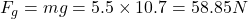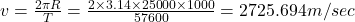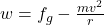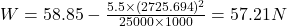## The acceleration due to gravity at the north pole of Neptune is approximately 10.7m/s2. Neptune has mass 1026kg and radius 25000km and rotat

Question

The acceleration due to gravity at the north pole of Neptune is approximately 10.7m/s2. Neptune has mass 1026kg and radius 25000km and rotates once around its axis in a time of about 16h.

Part A

What is the gravitational force on an object of mass 5.5kg at the north pole of Neptune?

Part B

What is the apparent weight of this same object at the Neptune’s equator? (Note that Neptune

in progress 0
2 months 2021-07-30T00:48:30+00:00 1 Answers 18 views 0

(A) Force on the object will be 58.85 N

(B) Apparent weight will be equal to 57.21 N

Explanation:

We have given mass of Neptune m = 1026 kg

Time period = 16 hour

We know that 1 hour = 3600 sec

So 16 hour = 16×86400 = 57600 sec

Acceleration due to gravity at north pole of Neptune(A) Mass of the object m = 5.5 kg

So gravitational force on the object(B) Velocity will be equal toApparent weight of the object will be equal to